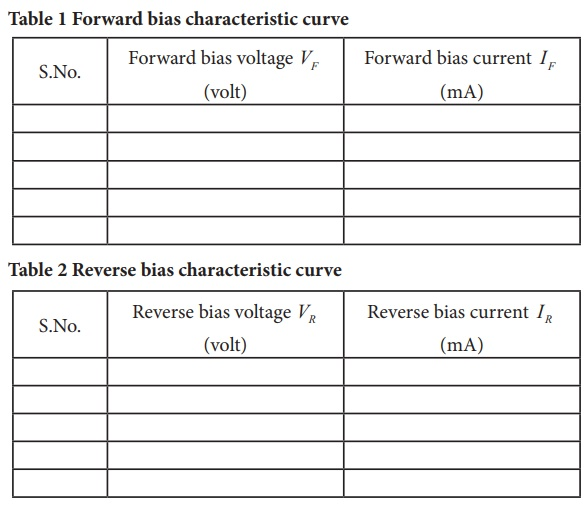Home | | Physics 12th Std | Voltage-Current Characteristics of a Zener Diode

# Voltage-Current Characteristics of a Zener Diode

To draw the voltage-current (V-I) characteristic curves of a Zener diode and to determine its knee voltage, forward resistance and reverse breakdown voltage.

VOLTAGE-CURRENT CHARACTERISTICS OF A ZENER DIODE

### AIM

To draw the voltage-current (V-I) characteristic curves of a Zener diode and to determine its knee voltage, forward resistance and reverse breakdown voltage.

### APPARATUS REQUIRED

Zener diode IZ5.6V, variable dc power supply (0 ŌĆō 15V), milli ammeter, volt meter, 470ŌĆå╬® resistance, and connecting wires.

### FORMULAwhere,

RF ŌåÆ Forward resistance of the diode (╬®)

ŌłåVF ŌåÆ The change in forward voltage (volt)

ŌłåIF ŌåÆ The change in forward current (mA)

### CIRCUIT DIAGRAMFigure (a) Zener diode and its symbol (The black colour ring denotes the negative terminal of the Zener diode)

Figure (b) Zener diode in forward biasFigure (c) Zener diode in reverse bias

Precaution

Care should be taken to connect the terminals of ammeter, voltmeter, dc power supply and the Zener diode with right polarity.

### i) Forward bias characteristics

┬Ę        In the forward bias, the P- region of the diode is connected to the positive terminal and N-re-gion to the negative terminal of the DC power supply.

┬Ę        The connections are given as per the circuit diagram.

┬Ę        The voltage across the diode can be varied with the help of the variable DC power supply.

┬Ę        The forward voltage (VF) across the diode is increased from 0.1V in steps of 0.1V up to 0.8V and the forward current (IF) through the diode is noted from the milli-ammeter. The read-ings are tabulated.

┬Ę        The forward voltage and the forward current are taken as positive.

┬Ę        A graph is drawn taking the forward voltage along the x-axis and the forward current along the y-axis.

┬Ę        The voltage corresponding to the dotted line in the forward characteristics gives the knee voltage or threshold voltage or turn-on voltage of the diode.

┬Ę        The slope in the linear portion of the forward characteristics is calculated. The reciprocal of the slope gives the forward resistance of the diode.

### ii) Reverse bias characteristics

┬Ę        In the reverse bias, the polarity of the DC power supply is reversed so that the P- region of the diode is connected to the negative terminal and N-region to the positive terminal of the DC power supply

┬Ę        The connections are made as given in the circuit diagram.

┬Ę        The voltage across the diode can be varied with the help of the variable DC power supply.

┬Ę        The reverse voltage (VR) across the diode is increased from 0.5V in steps of 0.5V up to 6V and the reverse current (IR) through the diode is noted from the milli-ammeter. The readings are tabulated.

┬Ę        Initially, the voltage is increased in steps of 0.5V. When the breakdown region is approxi-mately reached, then the input voltage may be raised in steps of, say 0.1V to find the break-down voltage.

┬Ę        The reverse voltage and reverse current are taken as negative.

┬Ę        A graph is drawn taking the reverse bias voltage along negative x-axis and the reverse bias current along negative y-axis.

┬Ę        In the reverse bias, Zener breakdown occurs at a particular voltage called Zener voltage VZ (~5.6 to 5.8V) and a large amount of current flows through the diode which is the character-istics of a Zener diode.

┬Ę        The breakdown voltage of the Zener diode is determined from the graph as shown.### OBSERVATION

Table 1 Forward bias characteristic curve

Table 2 Reverse bias characteristic curve### CALCULATION

(i) Forward resistance RF =

(ii) knee voltage =

(iii) The breakdown voltage of the Zener diode VZ = ----V

### RESULT

The V-I characteristics of the Zener diode are studied.

(i) Forward resistance RF =

(ii) knee voltage =

(iii) The breakdown voltage of the Zener diode VZ= ----V

### Practical Tips

┬Ę        The DC power supply voltage should to be increased only up to the specified range in the forward (0 ŌĆō 2ŌĆåV) and reverse (0 ŌĆō 15ŌĆåV) directions.

┬Ę        The voltage applied beyond this limit may damage the resistor or the diode.

┬Ę        Zener diode functions like an ordinary PN junction diode in the forward direction. Hence the forward characteristic is the same for both PN junction diode and Zener diode. Therefore, knee voltage and forward resistance can be determined as explained in the previous experiment.

┬Ę        Unlike ordinary PN junction diode, the reverse current in Zener diode is measured using milli-ammeter due to the large flow of current.

Tags : Physics Practical Experiment , 12th Physics : Practical
Study Material, Lecturing Notes, Assignment, Reference, Wiki description explanation, brief detail
12th Physics : Practical : Voltage-Current Characteristics of a Zener Diode | Physics Practical Experiment